# Fractional Part of a Circle Online Quiz

#### Excel add-in with c# VSTO and web

7 Lectures 1.5 hours

#### Word add-in using c# vsto and web types

8 Lectures 1 hours

#### The Ultimate Guide To Add Social Media Login to PHP Web Apps

17 Lectures 2 hours

Following quiz provides Multiple Choice Questions (MCQs) related to Fractional Part of a Circle. You will have to read all the given answers and click over the correct answer. If you are not sure about the answer then you can check the answer using Show Answer button. You can use Next Quiz button to check new set of questions in the quiz.Q 1 - How much of the circle is blank? Write your answer as a fraction in simplest form.### Explanation

Step 1:

Sum of fractional parts present = $\frac{1}{3}$ + $\frac{1}{6}$

= $\frac{2}{6}$ + $\frac{1}{6}$ = $\frac{\left ( 2+1 \right )}{6}$ = $\frac{3}{6}$ = $\frac{1}{2}$

Step 2:

Fractional part of blank = 1 − $\frac{1}{2}$ = $\frac{2}{2}$$\frac{1}{2} = \frac{\left ( 2-1 \right )}{2} = \frac{1}{2} Q 2 - How much of the circle is blank? Write your answer as a fraction in simplest form.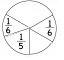### Answer : B ### Explanation Step 1: Sum of fractional parts present = \frac{1}{6} + \frac{1}{6} + \frac{1}{5} =\frac{2}{6} + \frac{1}{5} = \frac{10}{30} + \frac{6}{30} = \frac{16}{30} = \frac{8}{15} Step 2: Fractional part of blank = 1 − \frac{8}{15} = \frac{15}{15} - \frac{8}{15} = \frac{7}{15} Q 3 - How much of the circle is blank? Write your answer as a fraction in simplest form.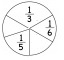### Answer : A ### Explanation Step 1: Sum of fractional parts present = \frac{1}{3} + \frac{1}{6} + \frac{1}{5} = \frac{10}{30} + \frac{5}{30} + \frac{6}{30} = \frac{21}{30} = \frac{7}{10} Step 2: Fractional part of blank = 1 - \frac{7}{10} = \frac{10}{10} - \frac{7}{10} = \frac{3}{10} Q 4 - How much of the circle is blank? Write your answer as a fraction in simplest form.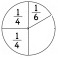### Answer : D ### Explanation Step 1: Sum of fractional parts present = \frac{1}{4} + \frac{1}{4} + \frac{1}{6} = \frac{3}{12} + \frac{3}{12} + \frac{2}{12} = \frac{8}{12} = \frac{2}{3} Step 2: Fractional part of blank = 1 - \frac{2}{3} = \frac{3}{3} - \frac{2}{3} = \frac{1}{3} Q 5 - How much of the circle is blank? Write your answer as a fraction in simplest form.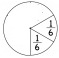### Answer : B ### Explanation Step 1: Sum of fractional parts present = \frac{1}{6} + \frac{1}{6} = \frac{\left ( 1+1 \right )}{6} = \frac{2}{6} = \frac{1}{3} Step 2: Fractional part of blank = 1 − \frac{1}{3} = \frac{3}{3} - \frac{1}{3} = \frac{2}{3} Q 6 - How much of the circle is blank? Write your answer as a fraction in simplest form.### Answer : C ### Explanation Step 1: Sum of fractional parts present = \frac{1}{6} + \frac{1}{5} = \frac{5}{30} + \frac{6}{30} = \frac{\left ( 5+6 \right )}{30} = \frac{11}{30} Step 2: Fractional part of blank = 1 − \frac{11}{30} = \frac{30}{30}$$\frac{11}{30}$ = $\frac{\left ( 30-11 \right )}{30}$ = $\frac{19}{30}$

Q 7 - How much of the circle is blank? Write your answer as a fraction in simplest form.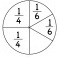### Explanation

Step 1:

Sum of fractional parts present = $\frac{1}{4}$ + $\frac{1}{4}$ + $\frac{1}{6}$ + $\frac{1}{6}$

=$\frac{2}{4}$ + $\frac{2}{6}$

= $\frac{6}{12}$ + $\frac{4}{12}$ = $\frac{10}{12}$ = $\frac{5}{6}$

Step 2:

Fractional part of blank = 1 − $\frac{5}{6}$ = $\frac{6}{6}$$\frac{5}{6} = \frac{1}{6} Q 8 - How much of the circle is blank? Write your answer as a fraction in simplest form.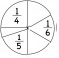### Answer : D ### Explanation Step 1: Sum of fractional parts present = \frac{1}{4} + \frac{1}{5} + \frac{1}{6} = \frac{15}{60} + \frac{12}{60} + \frac{10}{60} = \frac{\left ( 15+12+10 \right )}{60} = \frac{37}{60} Step 2: Fractional part of blank = 1 − \frac{37}{60} = \frac{60}{60}$$\frac{37}{60}$ = $\frac{\left ( 6-37 \right )}{60}$ = $\frac{23}{60}$

Q 9 - How much of the circle is blank? Write your answer as a fraction in simplest form.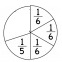### Explanation

Step 1:

Sum of fractional parts present = $\frac{1}{6}$ + $\frac{1}{6}$ + $\frac{1}{6}$ + $\frac{1}{5}$

=$\frac{3}{6}$ + $\frac{1}{5}$ = $\frac{15}{30}$ + $\frac{6}{30}$

= $\frac{\left ( 15+6 \right )}{30}$ = $\frac{21}{30}$ = $\frac{7}{10}$

Step 2:

Fractional part of blank = 1 − $\frac{7}{10}$ = $\frac{10}{10}$$\frac{7}{10} = \frac{\left ( 10-7 \right )}{10} = \frac{3}{10} Q 10 - How much of the circle is blank? Write your answer as a fraction in simplest form.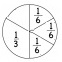### Answer : C ### Explanation Step 1: Sum of fractional parts present = \frac{1}{6} + \frac{1}{6} + \frac{1}{6} + \frac{1}{3} = \frac{3}{6} + \frac{1}{3} = \frac{6}{12} + \frac{4}{12} = \frac{\left ( 6+4 \right )}{12} = \frac{10}{12} = \frac{5}{6} Step 2: Fractional part of blank = 1− \frac{5}{6} = \frac{6}{6}$$\frac{5}{6}$ = $\frac{\left ( 6-5 \right )}{6}$ = $\frac{1}{6}$

fractional_part_of_circle.htm